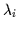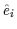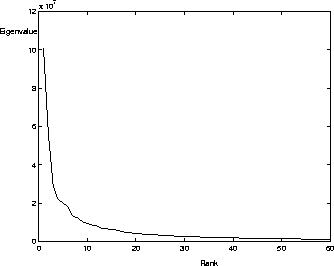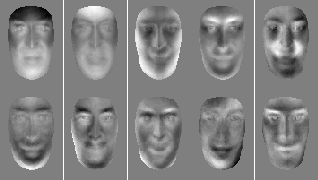Next: Encoding Face Images with Up: Karhunen-Loeve Decomposition for Statistical Previous: Karhunen-Loeve Decomposition for Statistical

## Computing Eigenvalues and Eigenvectors

The direct computation of eigenvalues and eigenvectors is a relatively straightforward operation for a symmetric matrix. We choose to solve the eigensystem using Jacobi transformations  (alternative techniques include Householder reductions followed by QR-QL and Hessenberg reductions followed by QR) . Unfortunately, direct methods are usually computationally expensive and require O(n3) operations.Thus, solving the eigensystem of the form Equationwould require O((n=7000)3) computations which would involve weeks of processing on an SGI Indy workstation. If we utilize the more efficient implementation found in Equationwe only need O((N=338)3)computations to find the eigenvectors. This requires only several hours of processing on an SGI Indy workstation.

If we wish to have a dynamic face recognition system that continuously enlarges D in real-time as it views new individuals, it is possible to recompute the PCA using more efficient iterative gradient search methods, such as the one proposed by Roseborough and Murase . However, we shall only compute the eigenvectors once for a dataset of N=338 faces and then reuse these eigenvectors on new faces which were not part of the original dataset matrix D. This approach is based on the assumption that a large enough initial training sample of 300+ mug-shot faces would populate the face-space'' cluster within the larger image-space'' quite adequately (i.e., densely and extensively enough). Thus, new faces will simply fall within the predetermined face-space region and hence are well approximated by the span of the eigenvectors that were previously generated .

Figureshows a plot of the magnitude of the eigenvalues versus their rank. The magnitude of an eigenvalue,, is equal to the variance in the data set that is spanned by its corresponding eigenvector,. Thus, it is obvious that higher-order eigenvectors account for less energy in the approximation of the data set since their eigenvalues have low magnitudes. We choose to truncate the set of eigenvectors to the first 60 vectors. This reduced set of eigenvectors accounts for enough face-variance to avoid excessively lossy compression. M=60 will be used throughout the experiments . The first 10 of our eigenvectors (also called eigenfaces since they are formed from face images) are shown in Figure.Next: Encoding Face Images with Up: Karhunen-Loeve Decomposition for Statistical Previous: Karhunen-Loeve Decomposition for Statistical
Tony Jebara
2000-06-23